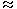# table chart aptitude questions

Study the following table and answer the questions.

Classification of 100 Students Based on the Marks Obtained by them in Physics and Chemistry in an Examination.

 Subject Marks out of 50 40 and above 30 and above 20 and above 10 and above 0 and above Physics 9 32 80 92 100 Chemistry 4 21 66 81 100 Average (Aggregate) 7 27 73 87 100
1. What is the different between the number of students passed with 30 as cut-off marks in Chemistry and those passed with 30 as cut-off marks in aggregate?
 A. 3 B. 4 C. 5 D. 6

Explanation:

Required difference

= (No. of students scoring 30 and above marks in Chemistry)

(Number of students scoring 30 and above marks in aggregate)

= 27 – 21

= 6.

2. If at least 60% marks in Physics are required for pursuing higher studies in Physics, how many students will be eligible to pursue higher studies in Physics?
 A. 27 B. 32 C. 34 D. 41

Explanation:

 We have 60% of 50 =60 x 50= 30. 100Required number

= No. of students scoring 30 and above marks in Physics

= 32

3. The percentage of number of students getting at least 60% marks in Chemistry over those getting at least 40% marks in aggregate, is approximately?
 A. 21% B. 27% C. 29% D. 31%

Explanation:

Number of students getting at least 60% marks in Chemistry

= Number of students getting 30 and above marks in Chemistry

= 21.

Number of students getting at least 40% marks in aggregate

= Number of students getting 20 and above marks in aggregate

= 73.

Required percentage
 =21 x 100% 73
= 28.77%29%.

4. The number of students scoring less than 40% marks in aggregate is?
 A. 13 B. 19 C. 20 D. 27

Explanation:

 We have 40% of 50 =40 x 50= 20. 100Required number

= Number of students scoring less than 20 marks in aggreagate

= 100 – Number of students scoring 20 and above marks in aggregate

= 100 – 73

= 27.

5. If it is known that at least 23 students were eligible for a Symposium on Chemistry, then the minimum qualifying marks in Chemistry for eligibility to Symposium would lie in the range?
 A. 40-45 B. 30-40 C. 20-30 D. Below 20